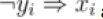# 2-Satisfiability(2-SAT) Problem in C/C++?

C++Server Side ProgrammingProgramming

#### C in Depth: The Complete C Programming Guide for Beginners

45 Lectures 4.5 hours

#### Practical C++: Learn C++ Basics Step by Step

Most Popular

50 Lectures 4.5 hours

#### Master C and Embedded C Programming- Learn as you go

66 Lectures 5.5 hours

Let f = (x1 ∨ y1) ∧ (x2 ∨ y2) ∧ ... ∧ (xn ∨ yn).

Problem: Is f satisfiable?

xi ∨ yi andandare all equivalent. So we are converting each of (xi ∨ yi) s into those two statements.

Now assume a graph with 2n vertices. In case of each of (xi∨yi) s two directed edges are added

• From ¬xi to yi

• From ¬yi to xi

f is not treated as satisfiable if both ¬xi and xi are in the same SCC (Strongly Connected Component)

Assume that f is treated as satisfiable. Now we want to provide values to each variable in order to satisfy f. It can be performed with a topological sort of vertices of the graph we construct. If ¬xi is after xi in topological sort, xi should be treated as FALSE. It should be treated as TRUE otherwise.

## Pseudo Code

func dfsFirst1(vertex v1):
marked1[v1] = true
for each vertex u1 adjacent to v1 do:
if not marked1[u1]:
dfsFirst1(u1)
stack.push(v1)
func dfsSecond1(vertex v1):
marked1[v1] = true
for each vertex u1 adjacent to v1 do:
if not marked1[u1]:
dfsSecond1(u1)
component1[v1] = counter
for i = 1 to n1 do:
for i = 1 to n1 do:
if not marked1[x[i]]:
dfsFirst1(x[i])
if not marked1[y[i]]:
dfsFirst1(y[i])
if not marked1[not x[i]]:
dfsFirst1(not x[i])
if not marked1[not y[i]]:
dfsFirst1(not y[i])
set all marked values false
counter = 0
flip directions of edges // change v1 -> u1 to u1 -> v1
while stack is not empty do:
v1 = stack.pop
if not marked1[v1]
counter = counter + 1
dfsSecond1(v1)
for i = 1 to n1 do:
if component1[x[i]] == component1[not x[i]]:
it is unsatisfiable
exit
if component1[y[i]] == component1[not y[i]]:
it is unsatisfiable
exit
it is satisfiable
exit